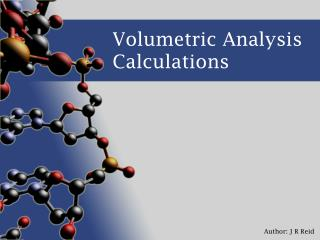DownloadDownload PresentationVolumetric Analysis Calculations

# Volumetric Analysis Calculations

Télécharger la présentation## Volumetric Analysis Calculations

- - - - - - - - - - - - - - - - - - - - - - - - - - - E N D - - - - - - - - - - - - - - - - - - - - - - - - - - -
##### Presentation Transcript

1. Volumetric Analysis Calculations

2. Example One: Jo has a solution of Hydrochloric acid (HCl) that she does not know the concentration of. She decides to use a standard solution of Sodium hydroxide (NaOH), 0.112molL-1, for the titration She uses a 15mL pipette to measure aliquots of NaOH into her flasks. She then titrates these with the HCl from the burette until the indicator turns from purple to colourless These are her results: Titres of HCl: 23.3mL, 22.8mL, 22.9mL, 22.7mL Balanced equation: → NaOH c = n = v = HCl c = n = v =

3. Example Two: • Jim has a solution of Sodium carbonate (Na2CO3), but the label is missing. To work out the concentration he decides to use a standard solution of Hydrochloric acid (HCl) with a concentration of 0.322molL-1. • He uses a 20mL pipette to measure aliquots of HCl into his flasks. He then titrates these with the Na2CO3 from the burette until the indicator turns from colourless to purple • These are his results: • Titres of Na2CO3 : 18.2mL, 18.2mL, 17.9mL, 18.3mL • Balanced equation: → • HCl c = n = v = • Na2CO3 c = n = v =

4. Example Three: • Jill has a bottle of vinegar (ethanoic acid solution – CH3COOH). The label says that it should have 3.1gL-1 of ethanoic acid in it. To work out the real concentration she decides to use a standard solution of Sodium hydroxide (NaOH) with a concentration of 1.22 x 10-2 molL-1. • She uses a 25mL pipette to measure aliquots of vinegar into her flasks. She then titrates these with the NaOH from the burette until the indicator turns from colourless to purple • These are her results: • Titres of NaOH : 22.1mL, 22.5mL, 22.6mL, 22.4mL • Balanced equation: → • NaOH c = n = v = • CH3COOH c = molL-1 gL-1 n = v =